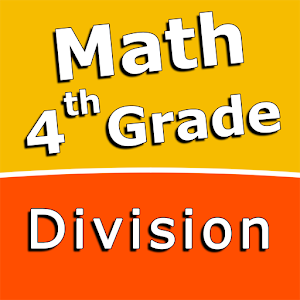\$2.99

# Android Giveaway of the Day - Fourth grade Math skills - Division

Practice and improve your math skills!
\$2.99 期限切れ
ユーザーの評価: 0

このgiveawayオファーはすでに期限が切れています。Fourth grade Math skills - Divisionが今紹介されています。

Intuitive interface powered by handwriting input and a choice of three fun and engaging mini games in addition to a regular math trainer mode make our app stand out from the crowd of generic math learning apps.

With Fourth grade Math skills - Division you can practice and improve the following math skills:
- Division facts to 12
- Divide two-digit numbers by one-digit numbers
- Divide three-digit numbers by one-digit numbers
- Divide three-digit numbers by two-digit numbers
- Divide four-digit numbers by one-digit numbers
- Divide four-digit numbers by two-digit numbers
- Divide numbers ending in zeroes by numbers up to 12

Choose between four different modes:
- Math Trainer
- Math Shot mini-game
- Math Gravity mini-game
- Math Trick Shot mini-game

Educational

## コメント Fourth grade Math skills - Division

Thank you for voting!